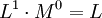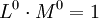# What is the dimension less physical quantity

A dimensionless size (correct description: Size of dimension one) is a physical quantity that is specified by a pure number without a unit of measurement can. There are several groups of dimensionless quantities:

• Proportions, these are physical quantities that are described by dividing two quantities of the same type of quantity. For the sake of clarity, units are usually used here too, but such a unit (auxiliary unit of measurement) does not correspond to any dimension.
• dimensionless relative indicators, these are descriptive parameters of a system, mostly in the sense of a material constant or a general description typical of the system
• pure numerical values, that is, mathematical quantities in physics that are described by numbers

### Examples

Examples of dimensionless quantities are

• Numbers
• Amounts of substance (base unit mole, dimension 1)
• Information in ratio units, percent, per thousand or ppm and the like
• Plane angles and solid angles (base units radians and steradian, dimension 1)
• Ratios, i.e. quotients from two dimensions of equal dimensions
• logarithmic ratios like Bel, Neper, Phon
• dimensionless key figures
• Probabilities
• Quantum numbers

Ratios is related sizeswhose relation is of the same size type (e.g. efficiency, the relation can then be a large number of sizes depending on the problem, and the numerical value of the efficiency depends on the reference variable)

The dimensionless key figures of fluid dynamics and thermohydraulics are important as intense sizeswhich can be used to predict the system behavior or which enable a comparison between different systems (of different dimensions). This includes, for example, the Reynolds number, which characterizes the flow quality (laminar / turbulent).

An example from another area is the Sommerfeld fine structure constant, which is composed of the elementary electrical charge, Planck's quantum of action and the speed of light. Their value is about 1/137. This constant was introduced by Arnold Sommerfeld in 1916 in order to be able to calculate the fine structure splitting of spectral lines caused by magnetic fields.

### Size designations according to DIN 5485

According to DIN 5485 Naming principles for physical quantities; Word compositions with adjectives and basic words is intended for dimensionless sizes:

• -number
• coefficient
• -factor
• -Degree
• quota
• -relationship
• -proportion of

In fact, in everyday scientific and technical life there are numerous names that do not follow these criteria, and dimensionless physical quantities can often, but not always, end with the ending -number detect. Also the ending -coefficient sometimes, but not always, denotes a dimensionless quantity. In the other case, it is related quantities (which are usually standardized to dimensions of mass, weight, volume and the like). Examples:

### Theoretical background

In metrology, a variable has dimension 1 if it is not assigned to any dimension of the respectively selected system of sizes. This is shown e.g. For example, when the corresponding dimension is represented as a power product of the base dimensions, each power is zero. In principle, there can be two reasons why a quantity does not belong to any dimension:

1. it is the quotient of two quantities of the same dimension
2. it has not yet been assigned to a dimension by definition

example: In a size system with only the two basic dimensions of length L. and mass M. the length has the dimension.

1. A quantity that is the quotient of two quantities of the dimension L. is defined, then always has the dimension L. / L. = 1.
2. A period of time would then initially have the dimension. However, an additional definition could make it dimensional, e.g. B. by assignment to the dimension L.3 or a newly introduced basic dimension of time T.

Since in principle (see relativity theory) time and length can be viewed as one and the same type of quantity (instead of as separate base quantities as e.g. in the SI system), one could also consider speed as a dimensionless ratio. However, this does not happen in the system of units used in practice: for historical reasons, because of the clarity and because of the "unwieldiness" of the conversion factor vacuum-light speed (1 s would correspond to approx. 300,000 km as a unit of length). In theoretical physics, however, the speed of light in a vacuum is often set equal to 1 (see natural units).

In principle, it depends on the basis chosen for a size system which derived sizes have which dimension, and thus also which sizes (apart from the quotients of identical sizes) have dimension 1. Thus, in electrostatic cgs systems, electrical capacitance and length are of the same dimension; every quotient of these quantities is therefore given the dimension 1.

The question of how to use the proportionality constants in the notation of physical laws is ultimately behind the choice of the basis of a size system.

Exclusively that coherent Dimensionless units of measurement in a system of units - of which there is only one for each type of quantity - dimensionless units also use the dimensionless units when calculating with the other units in the system Numerical value 1 at. Thus, within the SI system of units, 1 radian = 1 can be written, but not if the non-coherent dimensionless unit of measurement is used for the calculation.